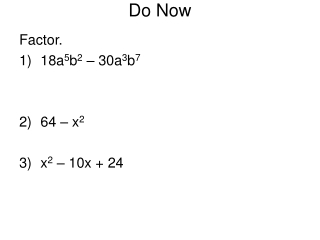DownloadDownload PresentationDo Now

# Do Now

Télécharger la présentation## Do Now

- - - - - - - - - - - - - - - - - - - - - - - - - - - E N D - - - - - - - - - - - - - - - - - - - - - - - - - - -
##### Presentation Transcript

1. Do Now Factor. • 18a5b2 – 30a3b7 • 64 – x2 • x2 – 10x + 24

2. Homework Solutions • (x + 3)(x + 1) • (m + 8)(m + 4) • (r – 2)(r – 1) • (x – 3)(x + 2) • (x – 7)(x + 3) • (x – 11)(x – 11) • (c – 6)(c + 2) • (p – 8)(p – 8) • x2 – 10x + 9 = (x – 9)(x – 1)

3. Practice Factor. • d2 – 8d + 15 • m2 + 10m + 9

4. 3) t2 – 8t – 20 4) c2 + 4c – 21

5. Rules For Factoring When you are factoring you should always follow these two rules: 1) Look for a GCF first!!!! 2) Check inside the ( ) to see if there is anything that can be factored more.

6. Factor completely. 3n2 + 18n + 15

7. 2) Factor completely. 4x3 – 20x2 – 96x

8. 3) Factor completely. 2t2 – 16t – 18

9. 4) Factor completely. 5c4d + 35c3d + 50c2d

10. 5) Factor completely. 2a3b2 – 22a2b2 + 56ab2

11. Homework Homework 2/1 Worksheet